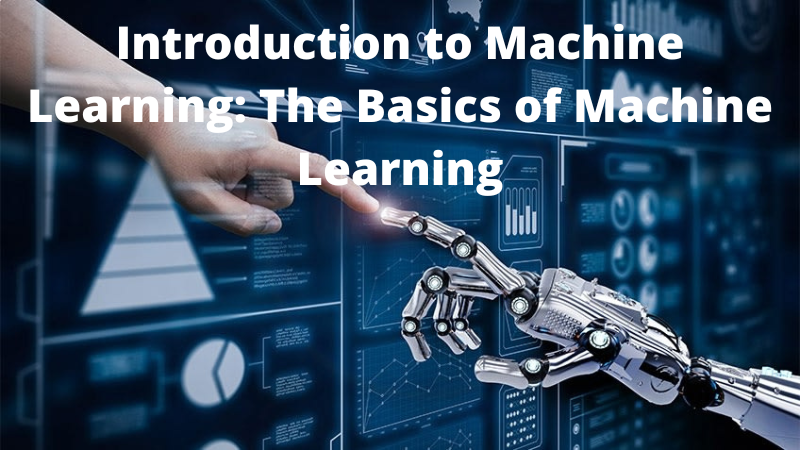Home Technology Introduction to Machine Learning: The Basics of Machine Learning
Technology

# Introduction to Machine Learning: The Basics of Machine Learning

by Niche Techy - 30 Aug 2022, Tuesday 124 Views Like (0)## What is machine learning?

Machine learning is a subset of artificial intelligence that focuses on providing computers with the ability to learn and improve from experience without being explicitly programmed. In other words, machine learning algorithms build models based on data that can be used to make predictions or recommendations. Machine learning algorithms are especially good at finding patterns in large sets of data. By identifying these patterns, they can provide accurate insights about how things work and how likely certain outcomes are. A classic example of this is predicting which ad to show you on Facebook when you�re browsing for something specific. You may have noticed ads for things you were looking for popping up even before you clicked on them! That�s because Facebook uses machine learning algorithms to identify trends and interests among its users, which then allows it to serve targeted ads.

## Classification vs. regression

In machine learning, there are two main types of problems: classification and regression. Classification is where you are trying to predict a label for a data point, and regression is where you are trying to predict a numeric value. An example of the first would be predicting whether an email message is spam or not spam. An example of the second would be predicting how many hours it will take someone to get from New York City to Washington D.C., based on certain factors such as traffic and driving style. There are plenty of other different types of machine learning algorithms, but these two categories cover the majority of what you'll see in practice.

## An exampl???????e classification problem

Classification problems are a type of machine learning problem where the goal is to predict a class label for data points. For example, you could use machine learning to build a system that predicts whether an email is spam or not. In this case, we would have two possible classes: spam and not spam. The goal would be to train our model so that it can correctly classify emails as either spam or not-spam with a high degree of accuracy. A typical way to do this is through training the model on a training set of labeled examples (i.e., emails labeled as spam or not). We then test the performance of our trained model on a testing set, which in this case would contain unlabeled examples (i.e., emails without labels). Finally, we calculate how many times out of 100 that our predicted classification matches what was actually labeled by humans in the training set; this number should ideally be close to 100%.

## Decision trees

Decision trees are flowchart-like structures in which a decision-making rule is determined by each question (e.g. is the fish predator or prey?). For each answer (for example, prey), there are two branches; if the fish is a predator, the other branch would lead to predators, if the fish is prey, the other branch would lead to prey. Leaf nodes represent a category. Paths from the root of the tree to the leaf represent the rule that decides what categories the fish should belong to.

## Random forests

In general, random forests are a type of machine learning algorithm which can be used for either regression or classification tasks. The predictor builds sets of decision trees. Every decision tree is trained on its own subset of the data. Accuracy of these methods is evaluated by judging their ability to accurately predict events. Predictive power is judged by measuring their predictive abilities by statistics such as variance reduction or ROC AUC (Receiver Operating Characteristic Area Under Curve). These determine how accurately the model can predict new examples, as opposed to examples it has seen before. One of the benefits of this approach is that it takes less training data than some other methods, like deep neural networks. Plus, it's shown that random forests often perform comparably to more computationally expensive models in many cases.

## Neural networks

Neural networks resemble the way the human brain operates in this way. Neurons in the input layer enter data. Neurons in the hidden layer process the data and extract features. There are neurons in the output layer that produce the final result.